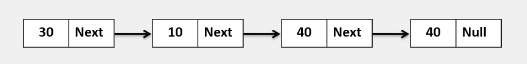# Golang program to insert a new node after the Kth node (K is not in the linked list)

## ExampleAdd node 15 after 50((K is not in the linked list)) value node.

Approach to solve this problem

Step 1 − Define a method that accepts the head of a linked list.

Step 3 − Iterate the given linked list.

## Example

Live Demo

package main
import (
"fmt"
)
type Node struct {
value int
next *Node
}
func NewNode(value int, next *Node) *Node{
var n Node
n.value = value
n.next = next
return &n
}
for temp != nil {
fmt.Printf("%d ", temp.value)
temp = temp.next
}
fmt.Println()
}
// Insert after Kth node(K is not in the linked list).
}
for temp != nil{
if temp.value == k{
newNode := NewNode(data, nil)
newNode.next = temp.next
temp.next = newNode
break
}
temp = temp.next
}
}
func main(){
head := NewNode(30, NewNode(10, NewNode(40, NewNode(40, nil))))
}
Input Linked list is: 30 10 40 40
Adding node after 50th value node, Linked List is: 30 10 40 40